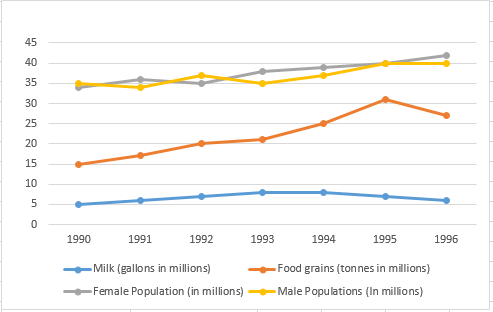# Line Graph Questions For CAT

0
2880

This post including the Important data interpretation line charts line graph and very most useful questions and tips and tricks

Line Graph Questions For CAT:

Download the CAT Verbal Ability PDFs/Ebooks and all Quantitative aptitude questions PDF.

Instructions:

The graph given below shows the quantity of milk and food grains consumed annually along with female and male population (in millions). Use the data to answer the questions that follow.Question 1:

When was the per capita production of milk least?

a) 1990

b) 1992

c) 1994

d) 1996

Question 2:

When was the per capita production of foodgrains most?

a) 1992

b) 1993

c) 1994

d) 1995

Question 3:

In which year was the difference between the percentage increase in the production of food grains and milk maximum?

a) 1993

b) 1994

c) 1995

d) 1996

Question 4:

If milk contains 320 calories and foodgrains contain 160 calories, in which year was the per capita consumption of calories highest?

a) 1993

b) 1994

c) 1995

d) 1996

Question 5:

If one gallon milk contains 120 g of a particular nutrient and one tonne of foodgrains contains 80 g of the same nutrient, in which year was the availability of this nutrient maximum?

a) 1993

b) 1994

c) 1995

d) 1996

Question 6:

Referring to the previous question, in which year was the per capita consumption of this nutrient highest?

a) 1993

b) 1994

c) 1995

d) 1996

Solutions:

The population is increasing steadily, but the milk produced decreased significantly in 1996.
Hence, the only two years we need to check for the least per capita milk production are the first year (1990) and 1996.
Milk produced in 1990 is 5 million gallons.
Total population in 1990 is 34+35 = 69 million.
Hence, per capita production of milk in 1990 is 0.072 gallon per person.
Milk produced in 1996 is 6 million gallons.
Total population in 1996 is 40+42 = 82 million.
Hence, per capita production of milk in 1996 is 0.073 gallon per person.
Hence, the year with the least per capita production of milk is 1990.

In this question the total population does not change much in the whole span. So the per capita food grain production will be maximum when the total foodgrain production is maximum. It happens in 1995.

The difference is maximum when one quantity is maximum and other quantity is minimum. The percentage increase in production of food grains is maximum in 1995 and the percentage production increase in production of milk is negative for 1995.

The consumption of milk is comparatively lesser than that of food grains and also the consumption of milk does not change by much during the given period.
Hence, the change in food grains is the key to estimate the calorie count.
We can see the spike in consumption of food grains in 1995. Hence, percapita consumption is highest in 1995.